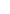## Power supplies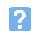1. The voltage at the input to IC1 will be approximately: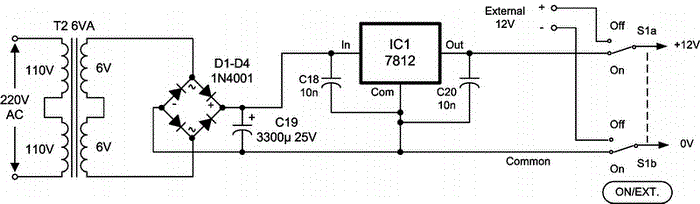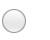a) 6 Vb) 12 Vc) 13.5 Vd) 16 V.2. In the diagram for Question 1, the function of IC1 is:a) a current amplifierb) a voltage amplifierc) a voltage regulatord) a miniature transformer.3. In the diagram for Question 1, the function of C19 is:a) a timing capacitorb) a coupling capacitorc) a reservoir capacitord) waveshaping capacitor.4. Which one of the following gives the frequency of the ripple produced by a full-wave bridge rectifier when fed from a 50Hz a.c. supply?a) 25 Hzb) 50 Hzc) 100 Hzd) 200 Hz.5. When a power supply is tested, the output voltage falls from 12V on no-load to 10 V when the load current is 1 A. Which one of the following gives the output resistance of the power supply?a) 0.5 ohmb) 1 ohmc) 2 ohmd) 10 ohm.6. Which one of the following is TRUE of the circuit shown below?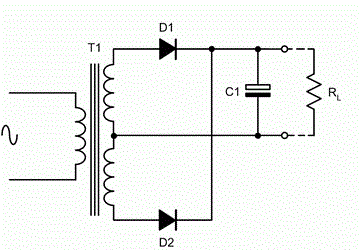a) The circuit acts as a voltage doublerb) The circuit acts as a voltage regulatoc) The circuit acts as a bi-phase rectifierd) The circuit acts as a half-wave rectfifier.7. The output voltage of a d.c. power supply falls from 20 V to 19 V when the a.c. mains supply voltage is reduced from 220 V to 200 V, Which one of the following gives the regulation of the supply?a) 0.5 %b) 1 %c) 2.5 %d) 5 %.8. The output voltage of a switched mode power supply can be regulated by controlling the:a) duty cycleb) input voltagec) output currentd) switching frequency.9. Most switched mode power supplies operate in the frequency range:a) below 3 kHzb) 3 kHz to 10 kHzc) 10 kHz to 30 kHzd) above 30 kHz.10. The advantages of a switched-mode power supply when compared with a conventional linear power supply include:a) low efficiency and compact sizeb) high efficiency and compact sizec) low noise generation and high efficiencyd) high noise generation and low efficiency.
This is more feedback!
This is the feedback!